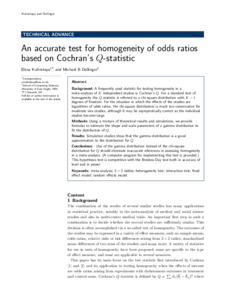# An accurate test for homogeneity of odds ratios based on Cochran's Q-statistic

Kulinskaya, Elena and Dollinger, Michael B. (2015) An accurate test for homogeneity of odds ratios based on Cochran's Q-statistic. BMC Medical Research Methodology, 15. ISSN 1471-2288Preview PDF (OR second bmc c) - Published Version Available under License Creative Commons Attribution. Download (838kB) | Preview

## Abstract

Background: A frequently used statistic for testing homogeneity in a meta-analysis of K independent studies is Cochran's Q. For a standard test of homogeneity the Q statistic is referred to a chi-square distribution with K - 1 degrees of freedom. For the situation in which the effects of the studies are logarithms of odds ratios, the chi-square distribution is much too conservative for moderate size studies, although it may be asymptotically correct as the individual studies become large. Methods: Using a mixture of theoretical results and simulations, we provide formulas to estimate the shape and scale parameters of a gamma distribution to t the distribution of Q. Results: Simulation studies show that the gamma distribution is a good approximation to the distribution for Q. Conclusions: : Use of the gamma distribution instead of the chi-square distribution for Q should eliminate inaccurate inferences in assessing homogeneity in a meta-analysis. (A computer program for implementing this test is provided.) This hypothesis test is competitive with the Breslow-Day test both in accuracy of level and in power.View Item